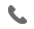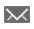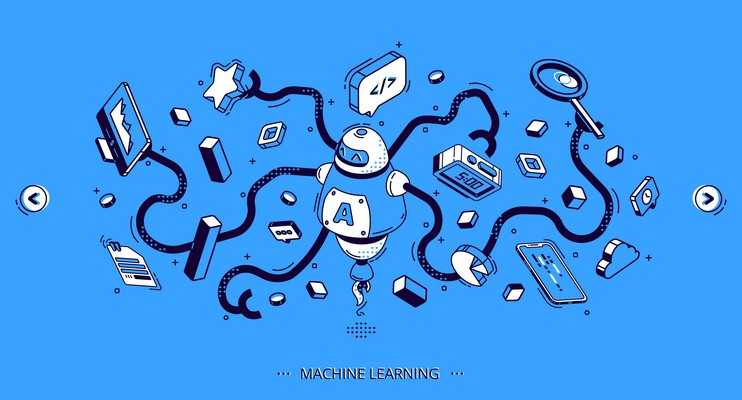•+44 7459 302492info@uplatz.com
• Register
Job Meter = High

### Machine Learning

25 Hours
Self-paced Training (pre-recorded videos)
USD 17 (USD 140)
Save 88% Offer ends on 30-Nov-2023652 Learners

Machine Learning is a data analytical method under Artificial Intelligence, used to automate the entire processing without the human intervention and with minimalistic code. It uses its algorithms in identifying patterns and making fast and efficient decisions. It has gained immense popularity in recent years with the adoption of Artificial Intelligence by almost every industry and in coming years, Machine Learning is going to be the future in every aspect.

Machine Learning basics starts with understanding the components involved in it which are (i)Representation, (ii)Evaluation and (iii)Optimization. There are different approaches to get started with the basics of Machine Learning, from decision trees to clustering layers of artificial neural networks, where the emphasis is mostly laid on different Machine Learning Algorithms.

In this Machine Learning Mastery course by Uplatz, you will be able to learn the basics and introduction to Machine Learning, Linear and Logistic Regression, Naïve Bayes, introduction to Deep Learning, Natural Language Processing and many more. Along with the theoretical explanation, you will also get hands-on exercises on different concepts of Machine Learning and its implementation.

---------------------------------------------------------------------------------------------------------

# Machine Learning

Course Details & Curriculum

Module 01 - Introduction to Machine Learning

1.1 Need of Machine Learning
1.2 Introduction to Machine Learning
1.3 Types of Machine Learning, such as supervised, unsupervised, and reinforcement learning, Machine Learning with Python, and the applications of Machine Learning

Module 02 - Supervised Learning and Linear Regression

2.1 Introduction to supervised learning and the types of supervised learning, such as regression and classification
2.2 Introduction to regression
2.3 Simple linear regression
2.4 Multiple linear regression and assumptions in linear regression
2.5 Math behind linear regression

Hands-on Exercise:

1. Implementing linear regression from scratch with Python
2. Using Python library Scikit-learn to perform simple linear regression and multiple linear regression
3. Implementing train–test split and predicting the values on the test set

Module 03 - Classification and Logistic Regression

3.1 Introduction to classification
3.2 Linear regression vs Logistic regression
3.3 Math behind logistic regression, detailed formulas, the logit function and odds, confusion matrix and accuracy, true positive rate, false positive rate, and threshold evaluation with ROCR

Hands-on Exercise:

1. Implementing logistic regression from scratch with Python
2. Using Python library Scikit-learn to perform simple logistic regression and multiple logistic regression
3. Building a confusion matrix to find out accuracy, true positive rate, and false positive rate

Module 04 - Decision Tree and Random Forest

4.1 Introduction to tree-based classification
4.2 Understanding a decision tree, impurity function, entropy, and understanding the concept of information gain for the right split of node
4.3 Understanding the concepts of information gain, impurity function, Gini index, overfitting, pruning, pre-pruning, post-pruning, and cost-complexity pruning
4.4 Introduction to ensemble techniques, bagging, and random forests and finding out the right number of trees required in a random forest

Hands-on Exercise:

1. Implementing a decision tree from scratch in Python
2. Using Python library Scikit-learn to build a decision tree and a random forest
3. Visualizing the tree and changing the hyper-parameters in the random forest

Module 05 - Naïve Bayes and Support Vector Machine

5.1 Introduction to probabilistic classifiers
5.2 Understanding Naïve Bayes and math behind the Bayes theorem
5.3 Understanding a support vector machine (SVM)
5.4 Kernel functions in SVM and math behind SVM

Hands-on Exercise:

1. Using Python library Scikit-learn to build a Naïve Bayes classifier and a support vector classifier

Module 06 - Unsupervised Learning

6.1 Types of unsupervised learning, such as clustering and dimensionality reduction, and types of clustering
6.2 Introduction to k-means clustering
6.3 Math behind k-means
6.4 Dimensionality reduction with PCA

Hands-on Exercise:

1. Using Python library Scikit-learn to implement k-means clustering
2. Implementing PCA (principal component analysis) on top of a dataset

Module 07 - Natural Language Processing and Text Mining (self-paced)

7.1 Introduction to Natural Language Processing (NLP)
7.2 Introduction to text mining
7.3 Importance and applications of text mining
7.4 How NPL works with text mining
7.5 Writing and reading to word files
7.6 Language Toolkit (NLTK) environment
7.7 Text mining: Its cleaning, pre-processing, and text classification

Hands-on Exercise:

1. Learning Natural Language Toolkit and NLTK Corpora
2. Reading and writing .txt files from/to a local drive
3. Reading and writing .docx files from/to a local drive

Module 08 - Introduction to Deep Learning

8.1 Introduction to Deep Learning with neural networks
8.2 Biological neural networks vs artificial neural networks
8.3 Understanding perception learning algorithm, introduction to Deep Learning frameworks, and TensorFlow constants, variables, and place-holders

Module 09 - Time Series Analysis (Self-paced)

9.1 What is time series? Its techniques and applications
9.2 Time series components
9.3 Moving average, smoothing techniques, and exponential smoothing
9.4 Univariate time series models
9.5 Multivariate time series analysis
9.6 ARIMA model and time series in Python
9.7 Sentiment analysis in Python (Twitter sentiment analysis) and text analysis

Hands-on Exercise:

1. Analyzing time series data
2. The sequence of measurements that follow a non-random order to recognize the nature of phenomenon
3. Forecasting the future values in the series

------------------------------------------------------------------------------------------------------